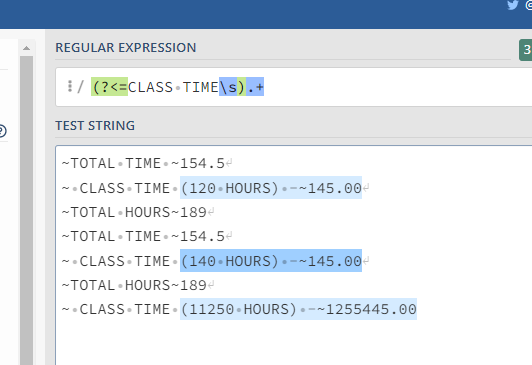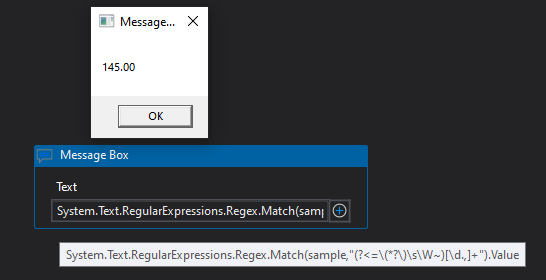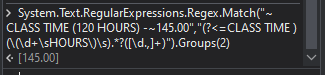# Regular expression issue

~TOTAL TIME ~154.5
~ CLASS TIME (120 HOURS) -~145.00
~TOTAL HOURS~189
This is the sample txt data, i want to get the value 145
i provided regex as (?<=CLASS TIME (120 HOURS)).* but this 120HOURS is not taking,
so any one can you please suggest how can i resolve this, (120HOURS),This value is dynamic.

@Chippy_Kolot ok wait

Try the below Regex :

``````(?<=CLASS TIME \(.*\)).*?([\d.,]+)
``````

You would need to access the Group 1 from the Match result to get only the number of hours`````` strText
@"~TOTAL TIME ~154.5
~ CLASS TIME (120 HOURS) -~145.00
~TOTAL HOURS~189"
strPattern
"(?<=CLASS TIME.*?~)[\d.,]+"
System.Text.RegularExpressions.Regex.Match(strText, strPattern).Value
"145.00"
``````

Along with CLASS TIME (120 HOURS) Need to be taken, because this thing can repeat with different time(eg:20HOURS)

@Chippy_Kolot Try this

``````(?<=CLASS TIME\s).+
``````Otherwise try this

``````System.Text.RegularExpressions.Regex.Match(sample,"(?<=\(*?\)\s\W~)[\d.,]+").Value
````````````System.Text.RegularExpressions.Regex.Match(YourString,"(?<=CLASS TIME )(\(\d+\sHOURS\)\s).*?([\d.,]+)").Groups(2)
``````

OutputRegards
Gokul

Hey!

Try this and let me know

``````(?<=CLASS TIME ).+
``````

The above regex will give you the (120 HOURS) -~145.00

Reference:

Regards,
NaNi